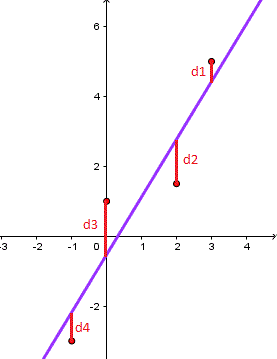# Linear Regression Problems with Solutions

Linear regression and modelling problems are presented along with their solutions at the bottom of the page. Also a linear regression calculator and grapher may be used to check answers and create more opportunities for practice.

## Review

If the plot of n pairs of data (x , y) for an experiment appear to indicate a "linear relationship" between y and x, then the method of least squares may be used to write a linear relationship between x and y.
The least squares regression line is the line that minimizes the sum of the squares (d1 + d2 + d3 + d4) of the vertical deviation from each data point to the line (see figure below as an example of 4 points).Figure 1. Linear regression where the sum of vertical distances d1 + d2 + d3 + d4 between observed and predicted (line and its equation) values is minimized. The least square regression line for the set of n data points is given by the equation of a line in slope intercept form:
y = a x + b

where a and b are given byFigure 2. Formulas for the constants a and b included in the linear regression .
• ### Problem 1

Consider the following set of points: {(-2 , -1) , (1 , 1) , (3 , 2)}
a) Find the least square regression line for the given data points.
b) Plot the given points and the regression line in the same rectangular system of axes.
• ### Problem 2

a) Find the least square regression line for the following set of data
{(-1 , 0),(0 , 2),(1 , 4),(2 , 5)}

b) Plot the given points and the regression line in the same rectangular system of axes.
• ### Problem 3

The values of y and their corresponding values of y are shown in the table below
 x 0 1 2 3 4 y 2 3 5 4 6

a) Find the least square regression line y = a x + b.
b) Estimate the value of y when x = 10.
• ### Problem 4

The sales of a company (in million dollars) for each year are shown in the table below.
 x (year) 2005 2006 2007 2008 2009 y (sales) 12 19 29 37 45

a) Find the least square regression line y = a x + b.
b) Use the least squares regression line as a model to estimate the sales of the company in 2012.

### Solutions to the Above Problems

1. a) Let us organize the data in a table.
x y x y x 2
-2 -1 2 4
1 1 1 1
3 2 6 9
?x = 2 ?y = 2 ?xy = 9 ?x2 = 14

We now use the above formula to calculate a and b as follows
a = (n?x y - ?x?y) / (n?x2 - (?x)2) = (3*9 - 2*2) / (3*14 - 22) = 23/38
b = (1/n)(?y - a ?x) = (1/3)(2 - (23/38)*2) = 5/19
b) We now graph the regression line given by y = a x + b and the given points.Figure 3. Graph of linear regression in problem 1.
2. a) We use a table as follows
x y x y x 2
-1 0 0 1
0 2 0 0
1 4 4 1
2 5 10 4
?x = 2 ?y = 11 ?x y = 14 ?x2 = 6

We now use the above formula to calculate a and b as follows
a = (n?x y - ?x?y) / (n?x2 - (?x)2) = (4*14 - 2*11) / (4*6 - 22) = 17/10 = 1.7
b = (1/n)(?y - a ?x) = (1/4)(11 - 1.7*2) = 1.9
b) We now graph the regression line given by y = ax + b and the given points.Figure 4. Graph of linear regression in problem 2.
3. a) We use a table to calculate a and b.
x y x y x 2
0 2 0 0
1 3 3 1
2 5 10 4
3 4 12 9
4 6 24 16
?x = 10 ?y = 20 ?x y = 49 ?x2 = 30

We now calculate a and b using the least square regression formulas for a and b.
a = (n?x y - ?x?y) / (n?x2 - (?x)2) = (5*49 - 10*20) / (5*30 - 102) = 0.9
b = (1/n)(?y - a ?x) = (1/5)(20 - 0.9*10) = 2.2
b) Now that we have the least square regression line y = 0.9 x + 2.2, substitute x by 10 to find the value of the corresponding y.
y = 0.9 * 10 + 2.2 = 11.2
4. a) We first change the variable x into t such that t = x - 2005 and therefore t represents the number of years after 2005. Using t instead of x makes the numbers smaller and therefore manageable. The table of values becomes.
 t (years after 2005) 0 1 2 3 4 y (sales) 12 19 29 37 45

We now use the table to calculate a and b included in the least regression line formula.
t y t y t 2
0 12 0 0
1 19 19 1
2 29 58 4
3 37 111 9
4 45 180 16
?x = 10 ?y = 142 ?xy = 368 ?x2 = 30

We now calculate a and b using the least square regression formulas for a and b.
a = (n?t y - ?t?y) / (n?t2 - (?t)2) = (5*368 - 10*142) / (5*30 - 102) = 8.4
b = (1/n)(?y - a ?x) = (1/5)(142 - 8.4*10) = 11.6
b) In 2012, t = 2012 - 2005 = 7
The estimated sales in 2012 are: y = 8.4 * 7 + 11.6 = 70.4 million dollars.